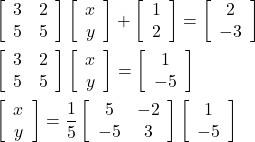## Solve the equation: [3 2 5 5] [x1 x2]+ [1 2]= [2 -3]

Question

Solve the equation:
[3 2
5 5]

[x1
x2]+
[1
2]=
[2
-3]

in progress 0
5 months 2021-09-05T11:27:03+00:00 1 Answers 0 views 0

1. 9514 1404 393

(x1, x2) = (3, -4)

Step-by-step explanation:

As with any 2-step linear equation, subtract the constant, then multiply by the inverse of the coefficient of the variable.Performing the multiplication of the matrix by the vector gives the solution.

x = ((5)(1) +(-2)(-5))/5 = 15/5 = 3

y = ((-5)(1) +(3)(-5))/5 = -20/5 = -4

Using your variables, x1, x2, the solution is …

(x1, x2) = (3, -4)